# Python Function

## Python Programming Training Certification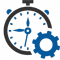Flexible Hours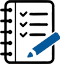100 Assignments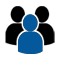Instructor Led online Training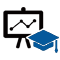50 LMS Access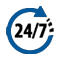24X7 Support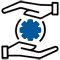100% Skill Level

### Enquire Now

4.9 out of 1000+ Ratings
Best Python Institute for Learning Python Course & Training, Live Project Training in Python with Django, Data Science and AI, Interview Assistance, Expert Coaching Trainers. Python Certification & Interview Assistance! Get free demo now!

### Course Overview

Python is one of the world’s top programming languages used today and Python training has become the most popular training across individuals. Training Basket’s Python Training & Certification course covers basic and advanced Python concepts and how to apply them in real-world applications.Python is a flexible and powerful open-source language that is easy to learn and consists of powerful libraries for data analysis and manipulation. Our Python training course content is curated by experts as per the standard Industry curriculum. The curriculum, coding challenges and real-life problems cover data operations in Python, strings, conditional statements, error handling, shell scripting, web scraping and the commonly used Python web framework Django. Take this Python training and certification course and become job-ready now.### Python Function

Functions are the most important aspect of an application. A function can be defined as the organized block of reusable code, which can be called whenever required.

Python allows us to divide a large program into the basic building blocks known as a function. The function contains the set of programming statements enclosed by {}. A function can be called multiple times to provide reusability and modularity to the Python program.

The Function helps to programmer to break the program into the smaller part. It organizes the code very effectively and avoids the repetition of the code. As the program grows, function makes the program more organized.

Python provide us various inbuilt functions like range() or print(). Although, the user can create its functions, which can be called user-defined functions.

There are mainly two types of functions.

• User-define functions – The user-defined functions are those define by the user to perform the specific task.
• Built-in functions – The built-in functions are those functions that are pre-defined in Python.
• In this tutorial, we will discuss the user define functions.

There are the following advantages of Python functions.

• Using functions, we can avoid rewriting the same logic/code again and again in a program.
• We can call Python functions multiple times in a program and anywhere in a program.
• We can track a large Python program easily when it is divided into multiple functions.
• Reusability is the main achievement of Python functions.
• However, Function calling is always overhead in a Python program.
Creating a Function

Python provides the def keyword to define the function. The syntax of the define function is given below.

Syntax:

• def my_function(parameters):
function_block
return expression

Let’s understand the syntax of functions definition.

• The def keyword, along with the function name is used to define the function.
• The identifier rule must follow the function name.
• A function accepts the parameter (argument), and they can be optional.
• The function block is started with the colon (:), and block statements must be at the same indentation.
• The return statement is used to return the value. A function can have only one return.
Function Calling

In Python, after the function is created, we can call it from another function. A function must be defined before the function call; otherwise, the Python interpreter gives an error. To call the function, use the function name followed by the parentheses.

Consider the following example of a simple example that prints the message “Hello World”.

• #function definition
def hello_world():
print(“hello world”)
# function calling
hello_world()

Output:

• hello world

The return statement

The return statement is used at the end of the function and returns the result of the function. It terminates the function execution and transfers the result where the function is called. The return statement cannot be used outside of the function.

Syntax

• return [expression_list]

It can contain the expression which gets evaluated and value is returned to the caller function. If the return statement has no expression or does not exist itself in the function then it returns the None object.

Consider the following example:

Example 1

• # Defining function
def sum():
a = 10
b = 20
c = a+b
return c
# calling sum() function in print statement
print(“The sum is:”,sum())

Output:

• The sum is: 30

In the above code, we have defined the function named sum, and it has a statement c = a+b, which computes the given values, and the result is returned by the return statement to the caller function.

Example 2 Creating function without return statement

• # Defining function
def sum():
a = 10
b = 20
c = a+b
# calling sum() function in print statement
print(sum())

Output:

• None

In the above code, we have defined the same function without the return statement as we can see that the sum() function returned the None object to the caller function.

Arguments in function

The arguments are types of information which can be passed into the function. The arguments are specified in the parentheses. We can pass any number of arguments, but they must be separate them with a comma.

Consider the following example, which contains a function that accepts a string as the argument.

Example 1

• #defining the function
def func (name):
print(“Hi “,name)
#calling the function
func(“Devansh”)

Output:

• Hi Devansh

Example 2

• #Python function to calculate the sum of two variables
#defining the function
def sum (a,b):
return a+b;

#taking values from the user
a = int(input(“Enter a: “))
b = int(input(“Enter b: “))

#printing the sum of a and b
print(“Sum = “,sum(a,b))

Output:

• Enter a: 10
Enter b: 20
Sum = 30

Call by reference in Python

In Python, call by reference means passing the actual value as an argument in the function. All the functions are called by reference, i.e., all the changes made to the reference inside the function revert back to the original value referred by the reference.

Example 1 Passing Immutable Object (List)

• #defining the function
def change_list(list1):
list1.append(20)
list1.append(30)
print(“list inside function = “,list1)

#defining the list
list1 = [10,30,40,50]

#calling the function
change_list(list1)
print(“list outside function = “,list1)

Output:

• list inside function = [10, 30, 40, 50, 20, 30]
list outside function = [10, 30, 40, 50, 20, 30]

Example 2 Passing Mutable Object (String)

• #defining the function
def change_string (str):
str = str + ” Hows you ”
print(“printing the string inside function :”,str)

string1 = “Hi I am there”

#calling the function
change_string(string1)

print(“printing the string outside function :”,string1)

Output:

• printing the string inside function : Hi I am there Hows you
printing the string outside function : Hi I am there

Types of arguments

There may be several types of arguments which can be passed at the time of function call.

• Required arguments
• Keyword arguments
• Default arguments
• Variable-length arguments

Required Arguments

Till now, we have learned about function calling in Python. However, we can provide the arguments at the time of the function call. As far as the required arguments are concerned, these are the arguments which are required to be passed at the time of function calling with the exact match of their positions in the function call and function definition. If either of the arguments is not provided in the function call, or the position of the arguments is changed, the Python interpreter will show the error.

Consider the following example.

Example 1

• def func(name):
message = “Hi “+name
return message
name = input(“Enter the name:”)
print(func(name))

Output:

• Enter the name: John
Hi John

Example 2

• #the function simple_interest accepts three arguments and returns the simple interest accordingly
def simple_interest(p,t,r):
return (p*t*r)/100
p = float(input(“Enter the principle amount? “))
r = float(input(“Enter the rate of interest? “))
t = float(input(“Enter the time in years? “))
print(“Simple Interest: “,simple_interest(p,r,t))

Output:

• Enter the principle amount: 5000
Enter the rate of interest: 5
Enter the time in years: 3
Simple Interest: 750.0

Example 3

• #the function calculate returns the sum of two arguments a and b
def calculate(a,b):
return a+b
calculate(10) # this causes an error as we are missing a required arguments b.

Output:

• TypeError: calculate() missing 1 required positional argument: ‘b’

Default Arguments

Python allows us to initialize the arguments at the function definition. If the value of any of the arguments is not provided at the time of function call, then that argument can be initialized with the value given in the definition even if the argument is not specified at the function call.

Example 1

• def printme(name,age=22):
print(“My name is”,name,”and age is”,age)
printme(name = “john”)

Output:

• My name is John and age is 22

Example 2

• def printme(name,age=22):
print(“My name is”,name,”and age is”,age)
printme(name = “john”) #the variable age is not passed into the function however the default value of age is considered in the function
printme(age = 10,name=”David”) #the value of age is overwritten here, 10 will be printed as age

Output:

• My name is john and age is 22
My name is David and age is 10

Variable-length Arguments (*args)

In large projects, sometimes we may not know the number of arguments to be passed in advance. In such cases, Python provides us the flexibility to offer the comma-separated values which are internally treated as tuples at the function call. By using the variable-length arguments, we can pass any number of arguments.

However, at the function definition, we define the variable-length argument using the *args (star) as *.

Consider the following example.

Example

• def printme(*names):
print(“type of passed argument is “,type(names))
print(“printing the passed arguments…”)
for name in names:
print(name)
printme(“john”,”David”,”smith”,”nick”)

Output:

• type of passed argument is
printing the passed arguments…
john
David
smith
nick

In the above code, we passed *names as variable-length argument. We called the function and passed values which are treated as tuple internally. The tuple is an iterable sequence the same as the list. To print the given values, we iterated *arg names using for loop.

Keyword arguments(**kwargs)

Python allows us to call the function with the keyword arguments. This kind of function call will enable us to pass the arguments in the random order.

The name of the arguments is treated as the keywords and matched in the function calling and definition. If the same match is found, the values of the arguments are copied in the function definition.

Consider the following example.

Example 1

• #function func is called with the name and message as the keyword arguments
def func(name,message):
print(“printing the message with”,name,”and “,message)

#name and message is copied with the values John and hello respectively
func(name = “John”,message=”hello”)

Output:

• printing the message with John and hello

Example 2 providing the values in different order at the calling

• #The function simple_interest(p, t, r) is called with the keyword arguments the order of arguments doesn’t matter in this case
def simple_interest(p,t,r):
return (p*t*r)/100
print(“Simple Interest: “,simple_interest(t=10,r=10,p=1900))

Output:

• Simple Interest: 1900.0

If we provide the different name of arguments at the time of function call, an error will be thrown.

Scope of variables

The scopes of the variables depend upon the location where the variable is being declared. The variable declared in one part of the program may not be accessible to the other parts.

In python, the variables are defined with the two types of scopes.

• Global variables
• Local variables

The variable defined outside any function is known to have a global scope, whereas the variable defined inside a function is known to have a local scope.

Consider the following example.

Example 1 Local Variable

• def print_message():
message = “hello !! I am going to print a message.” # the variable message is local to the function itself
print(message)
print_message()
print(message) # this will cause an error since a local variable cannot be accessible here.

Output:

• hello !! I am going to print a message.
File “/root/PycharmProjects/PythonTest/Test1.py”, line 5, in
print(message)
NameError: name ‘message’ is not defined

Example 2 Global Variable

• def calculate(*args):
sum=0
for arg in args:
sum = sum +arg
print(“The sum is”,sum)
sum=0
calculate(10,20,30) #60 will be printed as the sum
print(“Value of sum outside the function:”,sum) # 0 will be printed Output:

Output:

• The sum is 60
Value of sum outside the function: 0

### Recently Trained Students#### Jessica Biel

– Infosys

My instructor had sound Knowledge and used to puts a lot of effort that made the course as simple and easy as possible. I was aiming for with the help of the ZebLearn Online training imparted to me by this organization.#### Richard Harris

– ITC

I got my training from Zeblearn in the Python Certification Training, I would like to say that say he is one of the best trainers. He has not even trained me but also motivated me to explore more and the way he executed the project, in the end, was mind-blowing.

### Candidate’s Journey During Our Training Program#### Expert’s Advice & Selection of Module

Choosing the right type of module for the training is half the battle & Our Team of experts will help & guide you.#### Get Trained

Get Trained & Learn End to End Implementation from our Expert Trainer who are working on the same domain.#### Work on Projects

We Do make our student’s work on multiple case studies , scenario based tasks & projects in order to provide real-time exposure to them.#### Placements

We have a dedicated placement cell in order to provide placement assistance & relevant interviews to our candididates till selection